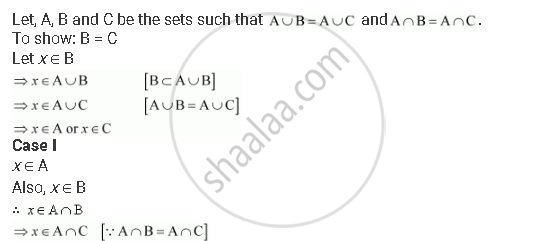# Let A, B and C Be the Sets Such that a ∪ B = a ∪ C and a ∩ B = a ∩ C. Show that B = C. - Mathematics

Sum

Let A, B and C be the sets such that A ∪ B = A ∪ C and A ∩ B = A ∩ C. show that B = C.

#### Solution∴ x ∈ A and x ∈ C

∴ x ∈ C

∴ B ⊂ C

Similarly, we can show that C ⊂ B.

∴ B = C

Concept: Subsets
Is there an error in this question or solution?

#### APPEARS IN

NCERT Class 11 Mathematics
Chapter 1 Sets
Exercise 1.7 | Q 3 | Page 26